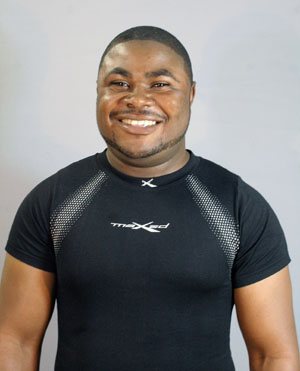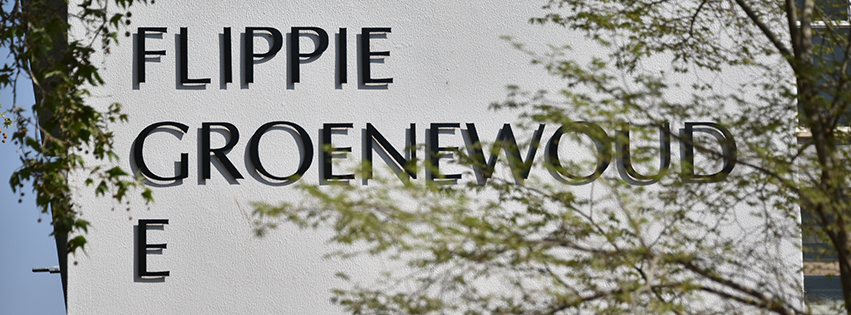Dr Abdon Atangana cements his research globally by solving fractional calculus problem
2014-12-03Dr Abdon Atangana

To publish 29 papers in respected international journals – and all of that in one year – is no mean feat. Postdoctoral researcher Abdon Atangana at the Institute for Groundwater Studies at the University of the Free State (UFS) reached this mark by October 2014, shortly before his 29th birthday.

His latest paper, ‘Modelling the Advancement of the Impurities and the Melted Oxygen concentration within the Scope of Fractional Calculus’, has been accepted for publication by the International Journal of Non-Linear Mechanics.

In previously-published research he solved a problem in the field of fractional calculus by introducing a fractional derivative called ‘Beta-derivative’ and its anti-derivative called ‘Atangana-Beta integral’, thereby cementing his research in this field.

Dr Atangana, originally from Cameroon, received his PhD in Geohydrology at the UFS in 2013. His research interests include:
• the theory of fractional calculus;
• modelling real world problems with fractional order derivatives;
• applications of fractional calculus;
• analytical methods for partial differential equations;
• analytical methods for ordinary differential equations;
• numerical methods for partial and ordinary differential equations; and
• iterative methods and uncertainties modelling.

Dr Atangana says that, “Applied mathematics can be regarded as the bridge between theory and practice. The use of mathematical tools for solving real world problems is as old as creation itself. As written in the book Genesis ‘And God saw the light, that it was good; and divided the light from the darkness’, the word division appears here as the well-known method of separation of variables, this method is usually employed to solve a class of linear partial differential equations”.

“A mathematical model is a depiction of a system using mathematical concepts and language. The procedure of developing a mathematical model is termed mathematical modelling. Mathematical models are used not only in natural sciences, but also in social sciences such as economics, psychology, sociology and political sciences. These models help to explain systems and to study the effects of different components, and to make predictions about behaviours.”

# FACULTY CONTACT

T: +27 51 401 2240 or humanities@ufs.ac.za